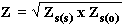# Measuring Characteristic Impedance and Effective Dielectric Constant of PCB tracks using a Vector Network Analyser

Introduction

After a PCB track width is calculated, it is a wise idea to check its Characteristic Impedance (Zo) before it is adopted for use in a project. It is also very useful to have some accurate data as benchmarks for checking the suitability of the many calculators that are available and a selection of sample tracks could be measured for this purpose. There is much information for using a Time Domain Reflectometer (TDR) in this measurement, but very little for using a Vector Network Analyser (VNA). One of the probable reasons for this is because it is not an easy measurement with a VNA. We have developed a VNA method which can show Zo over a wide bandwidth. This is of course difficult with a TDR, which has better resolution with a fast rise time (i.e. high frequencies, typically just greater than 1 GHz). Our method also shows the Effective Dielectric Constant (εeff), Electrical Delay, Velocity Factor and an approximation of the spot measurement frequencies.

Aim

i) To measure the Characteristic Impedance (Zo), Effective Dielectric Constant (εeff), Electrical Delay, Velocity Factor (Vf) over a wide frequency sweep for Microstrip tracks on FR4 PCB using a Vector Network Analyser (VNA) HP8753.

ii) To generate a graph of these results over a frequency bandwidth.

iii) To confirm the accuracy of these measurements using known information.

Samples

A sample PCB was manufactured, with three similar width tracks :-

i) SHORTED track 2 cm long.

ii) SHORTED track 22 cm long.

iii) OPEN track 22 cm long.

The tracks were separated by at least 0.5 cm of upper ground plane or 1.0 cm of blank PCB. Shorted ends were thoroughly grounded with multiple links through the double sided PCB. All ground planes were grounded with links at approximately every 5 mm.

All measurements were made via SMA connectors which were soldered directly onto the track and the ground plane. The board was tested on a wooden bench and the room was unscreened.

Method

The test method used was based on information gleaned from Transmission Lines and Networks by Walter C. Johnson, McGraw-Hill 1963 p115 Para 4.7 Measurement of the Characteristics of Lines.

Mr. Johnson states "A common problem is the determination of the characteristic impedance and propagation constant of a given transmission line which is physically available for measurement and test. These quantities can be found by the measurement of the input impedance of the line under two conditions: with the far end short-circuited, and with the far end open-circuited". He then arrives at the following formula.

W. C. Johnson Formula (4.49)Formula 1

He then discusses the measurement points.

"If the line is almost an odd number of quarter wavelengths long, the angle βl will be nearly an odd integer times π/2 radians; then Zs(s) will approach an open circuit and Zs(o) will approach a short circuit. This makes accurate measurements difficult. On the other hand, if the line is almost an even number of quarter wavelengths long, then Zs(o)will be very large and Zs(s) will be small. It is convenient to have both impedances of the same order of magnitude, and when the length of line can be selected at will, we can arrange to have tan βl approximately equal to 1/ tan βl. This occurs when the line is an odd number of eighth wavelengths long. If the over-all attenuation is high, the variation of impedance with length is not so violent, and so it is not necessary to select the length with care".

As we are measuring a very short length of track and the attenuation is low, it is important to select the measurement point with great care. After a few tests it became apparent that the best measurement point is indeed at the odd number of eighth wavelengths long. This corresponds to + 900 indication on the HP8753 VNA. It was also found that phase of the OPEN track was not accurate enough for calculating εeff, Electrical Delay and Velocity Factor. This is probably due to fringing or edge effect at the end of the open circuit track increasing the phase length as frequency increases. Therefore all phase references are made to the 22 cm SHORTED track.

1  2  3  >  Page

Technical Article Index